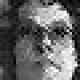02
Feb
11

GLSL Crosshatch

Straight port of
http://learningwebgl.com/blog/?p=2858

/*
Straight port of code from
http://learningwebgl.com/blog/?p=2858
*/

uniform sampler2D Texture;

void main()
{
float lum = length(texture2D(Texture, gl_TexCoord.xy).rgb);

gl_FragColor = vec4(1.0, 1.0, 1.0, 1.0);

if (lum < 1.00) {
if (mod(gl_FragCoord.x + gl_FragCoord.y, 10.0) == 0.0) {
gl_FragColor = vec4(0.0, 0.0, 0.0, 1.0);
}
}

if (lum < 0.75) {
if (mod(gl_FragCoord.x - gl_FragCoord.y, 10.0) == 0.0) {
gl_FragColor = vec4(0.0, 0.0, 0.0, 1.0);
}
}

if (lum < 0.50) {
if (mod(gl_FragCoord.x + gl_FragCoord.y - 5.0, 10.0) == 0.0) {
gl_FragColor = vec4(0.0, 0.0, 0.0, 1.0);
}
}

if (lum < 0.3) {
if (mod(gl_FragCoord.x - gl_FragCoord.y - 5.0, 10.0) == 0.0) {
gl_FragColor = vec4(0.0, 0.0, 0.0, 1.0);
}
}
}4 Responses to “GLSL Crosshatch”

1.1 Peter Boocock
February 3, 2011 at 1:12 am

That is a very useful link and interesting code; WebGL, definitely going to be gaining prominence.

2.2 George Toledo
July 29, 2011 at 5:47 am

I haven’t really fully thought it out yet, but looking at this got me thinking about how it would be really interesting to implement a dither in CI that would implement a shape at different size and densities depending on luminosity, so that low luminosity would get really small dots, and higher levels, big dots. It might look best to analyze the image into a voronoi, and then do a dot routine like I’m talking about, so that the placement of the dot dither looks less grid like. Not saying you should do this, just talking shop 🙂

•3 toneburst
September 3, 2011 at 9:53 pm

Interesting. I’ve been thinking about multi-pass pixellation type effects, with the grid0size varying for different areas. A lot of the 2D stuff I’ve been messing around with will probably be collated together into some kind of modular FX system at some point 🙂

a|x